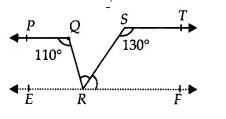Q

# In Fig. 6.31, if PQ || ST, ∠ PQR = 110° and ∠ RST = 130°, find ∠ QRS. [Hint : Draw a line parallel to ST through point R.]

4.  In Fig. 6.31, if PQ ST, PQR = 110° and RST = 130°, find QRS.
[Hint : Draw a line parallel to ST through point R.]Views

Draw a line EF parallel to the ST through R.
Since PQ || ST and ST || EF
EF || PQPQR = QRF =   (Alternate interior angles)
QRF = QRS + SRF .............(i)

Again, RST + SRF =  (Interior angles of two parallels ST and RF)
(RST = , given)

Thus, QRS =

Exams
Articles
Questions